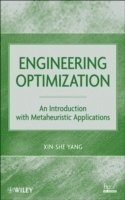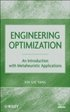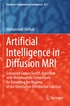Fler böcker inom
Format
Inbunden (Hardback)
Språk
Engelska
Antal sidor
376
Utgivningsdatum
2010-06-17
Upplaga
1
Förlag
John Wiley & Sons Inc
Medarbetare
Yang
Illustrationer
Illustrations
Dimensioner
236 x 160 x 23 mm
Vikt
640 g
Antal komponenter
1
Komponenter
ISBN
9780470582466

### Du kanske gillar# Engineering Optimization

An Introduction with Metaheuristic Applications

Inbunden Engelska, 2010-06-17
1269
Skickas inom 7-10 vardagar.
Fri frakt inom Sverige för privatpersoner.
Finns även som
1439
Modern optimization techniques are widely applicable to many applications, and metaheuristics form a class of emerging powerful algorithms for optimization. This book introduces state-of-the-art metaheuristic algorithms and their applications in optimization, using both MATLAB(R) and Octave allowing readers to visualize, learn, and solve optimization problems. It provides step-by-step explanations of all algorithms, case studies, real-world applications, and detailed references to the latest literature. It is ideal for researchers and professionals in mathematics, industrial engineering, and computer science, as well as students in computer science, engineering optimization, and computer simulation.

## Passar bra ihop

1.2. +
3.De som köpt den här boken har ofta också köpt Artificial Intelligence in Diffusion MRI av Mohammad Shehab (häftad).

## Kundrecensioner

Har du läst boken? Sätt ditt betyg »

## Övrig information

XIN-SHE YANG, PhD, is Senior Research Fellow in the Department of Engineering at Cambridge University (United Kingdom). The Editor-in-Chief of International Journal of Mathematical Modeling and Numerical Optimization (IJMMNO), Dr. Yang has published more than sixty journal articles in his areas of research interest, which include computational mathematics, metaheuristic algorithms, numerical analysis, and engineering optimization.

## Innehållsförteckning

List of Figures. Preface. Acknowledgments. Introduction. PART I Foundations of Optimization and Algorithms. 1.1 Before 1900. 1.2 Twentieth Century. 1.3 Heuristics and Metaheuristics. Exercises. 2 Engineering Optimization. 2.1 Optimization. 2.2 Type of Optimization. 2.3 Optimization Algorithms. 2.4 Metaheuristics. 2.5 Order Notation. 2.6 Algorithm Complexity. 2.7 No Free Lunch Theorems. Exercises. 3 Mathematical Foundations. 3.1 Upper and Lower Bounds. 3.2 Basic Calculus. 3.3 Optimality. 3.4 Vector and Matrix Norms. 3.5 Eigenvalues and Definiteness. 3.6 Linear and Affine Functions. 3.7 Gradient and Hessian Matrices. 3.8 Convexity. Exercises. 4 Classic Optimization Methods I. 4.1 Unconstrained Optimization. 4.2 Gradient-Based Methods. 4.3 Constrained Optimization. 4.4 Linear Programming. 4.5 Simplex Method. 4.6 Nonlinear Optimization. 4.7 Penalty Method. 4.8 Lagrange Multipliers. 4.9 Karush-Kuhn-Tucker Conditions. Exercises. 5 Classic Optimization Methods II. 5.1 BFGS Method. 5.2 Nelder-Mead Method. 5.3 Trust-Region Method. 5.4 Sequential Quadratic Programming. Exercises. 6 Convex Optimization. 6.1 KKT Conditions. 6.2 Convex Optimization Examples. 6.3 Equality Constrained Optimization. 6.4 Barrier Functions. 6.5 Interior-Point Methods. 6.6 Stochastic and Robust Optimization. Exercises. 7 Calculus of Variations. 7.1 Euler-Lagrange Equation. 7.2 Variations with Constraints. 7.3 Variations for Multiple Variables. 7.4 Optimal Control. Exercises. 8 Random Number Generators. 8.1 Linear Congruential Algorithms. 8.2 Uniform Distribution. 8.3 Other Distributions. 8.4 Metropolis Algorithms. Exercises. 9 Monte Carlo Methods. 9.1 Estimating p. 9.2 Monte Carlo Integration. 9.3 Importance of Sampling. Exercises. 10 Random Walk and Markov Chain. 10.1 Random Process. 10.2 Random Walk. 10.3 Levy Flights. 10.4 Markov Chain. 10.5 Markov Chain Monte Carlo. 10.6 Markov Chain and Optimisation. Exercises. PART II Metaheuristic Algorithms. 11 Genetic Algorithms. 11.1 Introduction. 11.2 Genetic Algorithms. 11.3 Implementation. Exercises. 12 Simulated Annealing. 12.1 Annealing and Probability. 12.2 Choice of Parameters. 12.3 SA Algorithm. 12.4 Implementation. Exercises. 13 Ant Algorithms. 13.1 Behaviour of Ants. 13.2 Ant Colony Optimization. 13.3 Double Bridge Problem. 13.4 Virtual Ant Algorithm. Exercises. 14 Bee Algorithms. 14.1 Behavior of Honey Bees. 14.2 Bee Algorithms. 14.3 Applications. Exercises. 15 Particle Swarm Optimization. 15.1 Swarm Intelligence. 15.2 PSO algorithms. 15.3 Accelerated PSO. 15.4 Implementation. 15.5 Constraints. Exercises. 16 Harmony Search. 16.1 Music-Based Algorithms. 16.2 Harmony Search. 16.3 Implementation. Exercises. 17 Firefly Algorithm. 17.1 Behaviour of Fireflies. 17.2 Firefly-Inspired Algorithm. 17.3 Implementation. Exercises. PART III Applications. 18 Multiobjective Optimization. 18.1 Pareto Optimality. 18.2 Weighted Sum Method. 18.3 Utility Method. 18.4 Metaheuristic Search. 18.5 Other Algorithms. Exercises. 19 Engineering Applications. 19.1 Spring Design. 19.2 Pressure Vessel. 19.3 Shape Optimization. 19.4 Optimization of Eigenvalues and Frequencies. 19.5 Inverse Finite Element Analysis. Exercises. Appendices. Appendix A: Test Problems in Optimization. Appendix B: Matlab(R) Programs. B.1 Genetic Algorithms. B.2 Simulated Annealing. B.3 Particle Swarm Optimization. B.4 Harmony Search. B.5 Firefly Algo